###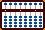Hexadecimal Numbers - Addition and Subtraction

##### Hex and Decimal Equivalents
 DEC 0 1 2 3 4 5 6 7 8 9 10 11 12 13 14 15 16 17 18 19 20 21 22 23 24 25 26 27 28 29 30 31 HEX 0 1 2 3 4 5 6 7 8 9 A B C D E F 10 11 12 13 14 15 16 17 18 19 1A 1B 1C 1D 1E 1F
##### Hexadecimal Values on a 2:5 Bead Suan Pan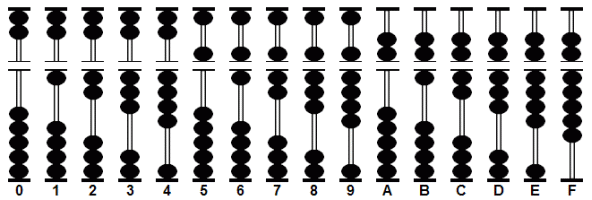When doing problems involving hexadecimals on a suan pan one must be aware of an important distinction. While hex A through hex F are all shown with two heaven beads touching the beam, there still exists the hex numbers 10, 11, 12, 13... etc.  Compare the two values A & 10; while both show variations of 10 on the abacus when added to another number each will yield a different answer. From the beginning it's important to distinguish between hex values that contain the expressions A through F and those using purely numeral expressions.

#### Complementary numbers

When calculating in the decimal numeral system (base 10) abacus operators make use of complementary numbers. Use the complementary numbers for hex (base 16) in the same way. In hexadecimal the complementary numbers make up hex 10 which converts to 16 in the decimal system.

 A & 6 B & 5 C & 4 D & 3 E & 2 F & 1 8 & 8 9 & 7

Rule: It may be a little confusing at first but there are rules regarding the heaven beads. In both addition and subtraction heaven beads take priority. In other words _use them first_ when they are available. Rather than get all muddled up trying to explain, it's better to see the examples below.

###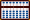Addition

##### Example 1a: A + 6 = 10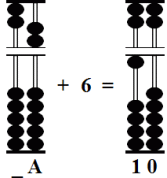##### Step 1: Add 6 to A

Add 6 to A. In order to continue we have to use a complementary number.

Solution: The complementary number for hex 6 is A. Subtract A and carry one bead to the left leaving the answer. Hex A + 6 = 10.

##### Example 1b: 10 + 6 = 16##### Step 1: Add 6 to 10

On the other hand adding hex 10 + 6 is a straight forward example. Add 6 to 10 leaving the answer 16.

##### Example 1c: A + A = 14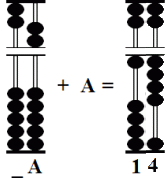##### Step 1: Add A to A

Add A to A. Use the complement.

Solution: The complementary number for hex A is 6. Subtract 6 from A leaving 4 and carry one bead to the left. Hex A + A = 14.

##### Example 2: D3 + 1A = ED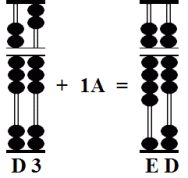##### Step 1: Add 1 to D  &  A to 3

Another straight forward example. There are no complementary number operations. Simply add 1 to D and A to to 3 leaving the answer. D3 + 1A = ED.

##### Example 3: F4 + 89 = 17D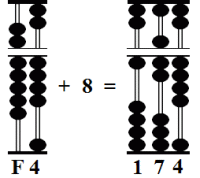##### Step 1: Add 8 to F4

Set F4. Add 8 to F. Use the complement.

Solution: The complementary number for 8 is 8. Subtract 8 and carry 1 to the left leaving 174.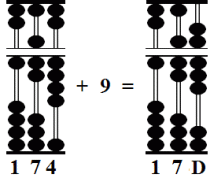##### Step 2: Add 9 to 4

Add 9 to 4. Because they are available make sure to use the top two heaven beads. Bring them down and subtract one earth bead leaving the answer. F4 + 89 = 17D.

##### Example 4: EA + AF = 199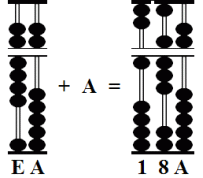##### Step 1: Add A to E

Set EA. Add A to E. Use the complement.

Solution: The complementary number for A is 6. Subtract 6 and carry 1 to the left leaving 18A on the frame.##### Step 2: Add F to A

Add F to A. Use the complement.

Solution: Subtract the complementary 1; do this by  moving up one heaven bead and four earth beads. Carry 1 to the left leaving the answer. EA + AF = 199.

##### Example 4: EC + BD = 1A9##### Step 1: Add B to E

Set EC. Add B to E. Use the complement.

Solution: The complementary number for B is 5. Subtract 5 and carry 1 to the left leaving 19C on the frame.##### Step 2: Add D to C

Add D to C. Use the complement.

Solution: Subtract the complementary 3; do this by  moving up one heaven bead and two earth beads. Carry 1 to the left. Do this by bringing down the heaven bead and subtracting 4 leaving the answer. EC + BD = 1A9.

###Subtraction

##### Example 1a: A - 6 = 4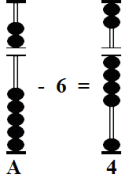##### Step 1: Subtract 6 from A

Subtract 6 from A. Make use of the heaven beads. Subtract A and make up the difference by adding four earth beads to the frame. This leaves the answer. A - 6 = 4

##### Example 1b: 10 - 6 = A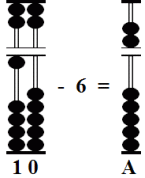##### Step 1: Subtract 6 from 10

Subtract 6 from 10. Use the complement.

Solution: The complementary number for 6 is A. Subtract 1 from the tens rod and add A to the unit rod leaving the answer. 10 - 6 = A

##### Example 2: 63 - 5B = 8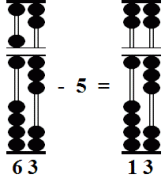##### Step 1: Subtract 5 from 6

Set 63. Subtract 5 from 6 on the tens rod  leaving 13.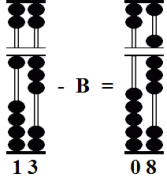##### Step 2: Subtract B from 3

Subtract B from 3 on the units rod. Use the complement.

Solution: Subtract 1 from the tens rod and add the complementary 5 leaving the answer. 63 - 5B = 8

##### Example 3: D4 - A9 = 2B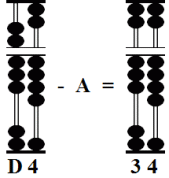##### Step 1: Subtract A from the tens rod

Set D4. Subtract A from from the tens rod leaving 34.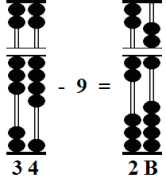##### Step 2: Subtract 9 from 4

Subtract 9 from 4 on the units rod. Use the complement.

Solution: Subtract 1 from the tens rod and to the units rod add the complementary 7. Because they are available make use of the heaven beads. Add the two heaven beads and subtract 3 leaving the answer. D4 - A9 = 2B

##### Example 4: 99 - 5C = 3D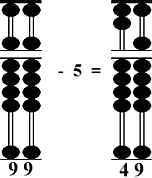##### Step 1: Subtract A from D

Set 99. Subtract 5 from 9 on the tens rod leaving 49.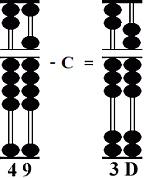##### Step 2: Subtract 9 from 4

Subtract C from 9 on the units rod. Use the complement.

Solution: Subtract 1 from the tens rod and to the units rod add the complementary 4. Because it is available make use of the heaven bead. Add the heaven bead and subtract 1 leaving the answer. 99 - 5C = 3D

REFERENCES: